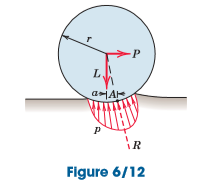Courses

# Rolling Resistance Mechanical Engineering Notes | EduRev

## Mechanical Engineering : Rolling Resistance Mechanical Engineering Notes | EduRev

The document Rolling Resistance Mechanical Engineering Notes | EduRev is a part of the Mechanical Engineering Course Engineering Mechanics.
All you need of Mechanical Engineering at this link: Mechanical Engineering

Rolling Resistance

Deformation at the point of contact between a rolling wheel and its supporting surface introduces a resistance to rolling, which we mention only brieﬂy. This resistance is not due to tangential friction forces and therefore is an entirely different phenomenon from that of dry friction.

To describe rolling resistance, we consider the wheel shown in Fig. 6/12 under the action of a load L on the axle and a force P applied at its center to produce rolling. The deformation of the wheel and supporting surfaces as shown is greatly exaggerated. The distribution of pressure p over the area of contact is similar to the distribution shown. The resultant R of this distribution acts at some point A and must pass through the wheel center for the wheel to be in equilibrium. We ﬁnd the force P necessary to maintain rolling at constant speed by equating the moments of all forces about A to zero. This gives usP=a/r L =μr

where the moment arm of P is taken to be r. The ratio ur =a/r is called the coefﬁcient of rolling resistance. This coefﬁcient is the ratio of resisting force to normal force and thus is analogous to the coefﬁcient of static or kinetic friction. On the other hand, there is no slippage or impending slippage in the interpretation of ur.

Because the dimension a depends on many factors which are difﬁcult to quantify, a comprehensive theory of rolling resistance is not available. The distance a is a function of the elastic and plastic properties of the mating materials, the radius of the wheel, the speed of travel, and the roughness of the surfaces. Some tests indicate that a varies only slightly with wheel radius, and thus a is often taken to be independent of the rolling radius. Unfortunately, the quantity a has also been called the coefﬁcient of rolling friction in some references. However, a has the dimension of length and therefore is not a dimensionless coefﬁcient in the usual sense.

Offer running on EduRev: Apply code STAYHOME200 to get INR 200 off on our premium plan EduRev Infinity!

## Engineering Mechanics

25 videos|71 docs|39 tests

,

,

,

,

,

,

,

,

,

,

,

,

,

,

,

,

,

,

,

,

,

;